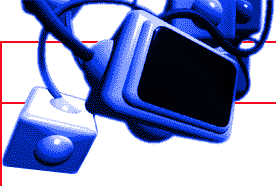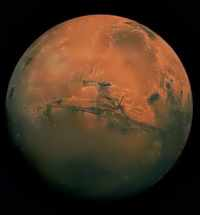EXPLORING MARS Action-pak Mathematics This Module has some applied mathematics problems to solve. They use simple multiplication and division, provide some problems using circles and a project associated with the Martian calendar. Gravity Mars is smaller than Earth and therefore exerts a lower gravitational pull on objects on its surface than does the Earth under the same conditions. As a simple exercise in percentages, multiplication and decimals, calculate what objects would weigh on Mars and other celestial bodies. The table below gives a gravitational G- Force for several bodies. Tasks a) Express your weight on Earth and Mars in Newtons. b) Work out what the value of g, the force due to gravity, is on Mars. c) Write a simple spreadsheet or computer program to calculate weights on celestial objects, using the gravity data supplied. d) Now think about athletics and other sports. How high would an athelete be able to jump on Mars. Is there likely to be a direct correlation, or do other effects come into play. Orbits The 16th Century scientist Johannes Kepler proved that objects orbit in an elliptical fashion, and while Kepler's laws may be introduced here, it is probably best to consider circular orbits, which are just a special case of Kepler. Many bodies in the Solar System, such as the Earth are in nearly circular orbits, anyway. Mars, though, is an exception. Its orbital eccentricity is nearly six times that of Earth, and varies from 206 to 249 million kilometres. Circular orbits can be used to demonstrate many of the basics of circular measure and calculation . TASK 1: The Orbiting Spacecraft a) A spacecraft orbits Mars at a height of 400 km . How far does it travel in one orbit to the nearest kilometre? b) The same spacecraft is orbiting at 18,000 kph, how long does it take to make one orbit (nearest second)? Mars has a diameter of 6800 km. TASK 2: Mars and Earth in Orbit It takes radio messages 4 minutes and 20 seconds to reach Earth from Mars, when Mars and Earth are at their closest. How long does it take for a radio signal to reach Earth when thy are at their farthest point from each other? Earth orbits the Sun at a distance of 150,000,000 km Mars orbits the Sun at a distance of 228,000,000 km You can work it out from these data. Is the answer, to the nearest minute:- a) 11 minutes, b) 21 minutes, c) 16 minutes, d) 24 minutes, e) 13 minutes ? TASK 3: Area of MarsMars Calendar The name sol is used for a Mars day. It is 24 hours 37 minutes 22 seconds long. The Martian year is 687 Earth days long. a) If we wanted to create a sol of 24 Martian hours, how long would it be to the nearest Earth second? b) How long is a Martian year in Earth years? c) Work out how many sols there are in a Martian year? Project Work Devise a calendar for the Martian year. Name the months and determine how many sols there should be in each month. Things to consider: Do you need Martian weeks and if so, how long are they going to be, in sols? Do you need twelve months in a year or should there be more months - or fewer? Bear in mind the seasons, and how long they will be. You could have a theme for the names of the months. The students should justify their reasoning. Shadow Effects, Distance and Size Measurement Explaining mathematical concepts is always easier and makes more of a lasting impression a) if a practical purpose can be demonstrated b) if a practical demonstration, hopefully interactive, can be given. You can use shadows to estimate the size of objects, and introduce some basic concepts of trigonometry, including sine, cosines and tangent relationships. Shadows of objects in sunshine can be used to tell us the size of objects. If we measure the projection of a shadow on the ground we can tell how tall, for instance, a building is, by using relating the shadow length to the angle of the Sun. This is obviously related to the time of day, the time of year and where on the planet we are doing the measurements. The greek astronomer Eratosthenes worked this out and from the information was able to estimate the size of the Earth. Eratosthenes of Cyrene 276BC -195BC: Eratosthenes served as chief librarian of the museum at Alexandria. He made the earliest recorded attempt to measure the size of the Earth in about 235BC. The story, probably apocraphal (though he obviously did some calculation), is as follows. He assumed the light beams from the Sun were parallel at the Earth - a fair assumption. He knew of a place in Syene (Aswan), some 800 km due south of Alexandria, where the sun shone vertically down a wellshaft on only one day a year, at noon - Midsummer's day. This implied to him that on this date, at this location the sun was directly overhead at 0 deg. He thought that if the Earth were curved, then at Alexandria, on the same day, at the same time, if a shadow were cast then it would imply curvature of the Earth's surface. Also, by measuring the length of the shadow and the height of the object casting it - simple post - he could calculate the angle of the rays in degrees. By dividing the distance to Aswan by this gave the distance for 1 deg of arc across the Earth's surface, and multiplying this result by 360 degrees would give the circumference of the Earth. This he did and came up with a result that wasn't bettered until the late C18 - 38400km. Aswan lies on the Tropic of Cancer and on Midsummer's day the Sun is precisely, vertically, overhead at noon. He originated a mathematical system, known as Eratosthenes' sieve, for determining prime numbers, and determined the inclination of the ecliptic to the celestial equator. Have your students check out Eratosthenes. For simple shadow casting experiments you can start with torch, a stick (or a pile of books or anything tall!), a protractor and a tape measure. You can then make measurements that relate angles with heights and lengths. The students can plot the results on graphs. Versions of this are suitable for all age bands. With younger children, for instance, the idea of light travelling in straight lines and the elongations of shadows at low angle of the Sun (or torch) are all that need to be introduced. Go to answers The Site uses as far as possible the mathematical conventions of the SI System, which has been adopted by over 90% of countries. Figures and values are also given in many cases, in non-SI units but these should be considered as approximate conversions, and not true values. Click here to return to the Exploring Mars Lessons IndexTeachers' Index | Home | Credits | Legal | About Us | Contact Details

© 1999 Satellite Events Enterprises Inc.